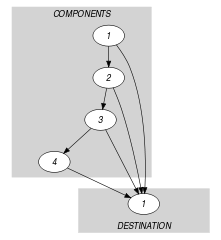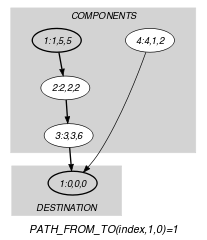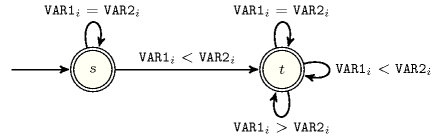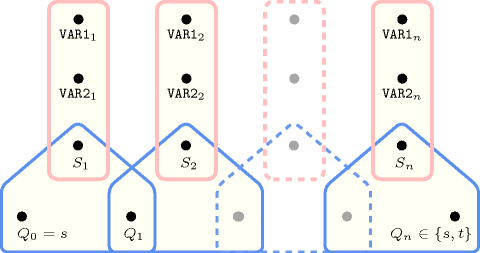## 5.232. lex_lesseq

Origin

CHIP

Constraint

$\mathrm{𝚕𝚎𝚡}_\mathrm{𝚕𝚎𝚜𝚜𝚎𝚚}\left(\mathrm{𝚅𝙴𝙲𝚃𝙾𝚁}\mathtt{1},\mathrm{𝚅𝙴𝙲𝚃𝙾𝚁}\mathtt{2}\right)$

Synonyms

$\mathrm{𝚕𝚎𝚡𝚎𝚚}$, $\mathrm{𝚕𝚎𝚡}_\mathrm{𝚌𝚑𝚊𝚒𝚗}$, $\mathrm{𝚛𝚎𝚕}$, $\mathrm{𝚕𝚎𝚜𝚜𝚎𝚚}$, $\mathrm{𝚕𝚎𝚚}$, $\mathrm{𝚕𝚎𝚡}_\mathrm{𝚕𝚎𝚚}$.

Arguments
 $\mathrm{𝚅𝙴𝙲𝚃𝙾𝚁}\mathtt{1}$ $\mathrm{𝚌𝚘𝚕𝚕𝚎𝚌𝚝𝚒𝚘𝚗}\left(\mathrm{𝚟𝚊𝚛}-\mathrm{𝚍𝚟𝚊𝚛}\right)$ $\mathrm{𝚅𝙴𝙲𝚃𝙾𝚁}\mathtt{2}$ $\mathrm{𝚌𝚘𝚕𝚕𝚎𝚌𝚝𝚒𝚘𝚗}\left(\mathrm{𝚟𝚊𝚛}-\mathrm{𝚍𝚟𝚊𝚛}\right)$
Restrictions
 $\mathrm{𝚛𝚎𝚚𝚞𝚒𝚛𝚎𝚍}$$\left(\mathrm{𝚅𝙴𝙲𝚃𝙾𝚁}\mathtt{1},\mathrm{𝚟𝚊𝚛}\right)$ $\mathrm{𝚛𝚎𝚚𝚞𝚒𝚛𝚎𝚍}$$\left(\mathrm{𝚅𝙴𝙲𝚃𝙾𝚁}\mathtt{2},\mathrm{𝚟𝚊𝚛}\right)$ $|\mathrm{𝚅𝙴𝙲𝚃𝙾𝚁}\mathtt{1}|=|\mathrm{𝚅𝙴𝙲𝚃𝙾𝚁}\mathtt{2}|$
Purpose

$\mathrm{𝚅𝙴𝙲𝚃𝙾𝚁}\mathtt{1}$ is lexicographically less than or equal to $\mathrm{𝚅𝙴𝙲𝚃𝙾𝚁}\mathtt{2}$. Given two vectors, $\stackrel{\to }{X}$ and $\stackrel{\to }{Y}$ of $n$ components, $〈{X}_{0},\cdots ,{X}_{n-1}〉$ and $〈{Y}_{0},\cdots ,{Y}_{n-1}〉$, $\stackrel{\to }{X}$ is lexicographically less than or equal to $\stackrel{\to }{Y}$ if and only if $n=0$ or ${X}_{0}<{Y}_{0}$ or ${X}_{0}={Y}_{0}$ and $〈{X}_{1},\cdots ,{X}_{n-1}〉$ is lexicographically less than or equal to $〈{Y}_{1},\cdots ,{Y}_{n-1}〉$.

Example
 $\left(〈5,2,3,1〉,〈5,2,6,2〉\right)$ $\left(〈5,2,3,9〉,〈5,2,3,9〉\right)$

The $\mathrm{𝚕𝚎𝚡}_\mathrm{𝚕𝚎𝚜𝚜𝚎𝚚}$ constraints associated with the first and second examples hold since:

• Within the first example $\mathrm{𝚅𝙴𝙲𝚃𝙾𝚁}\mathtt{1}=〈5,2,3,1〉$ is lexicographically less than or equal to $\mathrm{𝚅𝙴𝙲𝚃𝙾𝚁}\mathtt{2}=〈5,2,6,2〉$.

• Within the second example $\mathrm{𝚅𝙴𝙲𝚃𝙾𝚁}\mathtt{1}=〈5,2,3,9〉$ is lexicographically less than or equal to $\mathrm{𝚅𝙴𝙲𝚃𝙾𝚁}\mathtt{2}=〈5,2,3,9〉$.

Typical
 $|\mathrm{𝚅𝙴𝙲𝚃𝙾𝚁}\mathtt{1}|>1$ $\bigvee \left(\begin{array}{c}|\mathrm{𝚅𝙴𝙲𝚃𝙾𝚁}\mathtt{1}|<5,\hfill \\ \mathrm{𝚗𝚟𝚊𝚕}\left(\left[\mathrm{𝚅𝙴𝙲𝚃𝙾𝚁}\mathtt{1}.\mathrm{𝚟𝚊𝚛},\mathrm{𝚅𝙴𝙲𝚃𝙾𝚁}\mathtt{2}.\mathrm{𝚟𝚊𝚛}\right]\right)<2*|\mathrm{𝚅𝙴𝙲𝚃𝙾𝚁}\mathtt{1}|\hfill \end{array}\right)$ $\bigvee \left(\begin{array}{c}\mathrm{𝚖𝚊𝚡𝚟𝚊𝚕}\left(\left[\mathrm{𝚅𝙴𝙲𝚃𝙾𝚁}\mathtt{1}.\mathrm{𝚟𝚊𝚛},\mathrm{𝚅𝙴𝙲𝚃𝙾𝚁}\mathtt{2}.\mathrm{𝚟𝚊𝚛}\right]\right)\le 1,\hfill \\ 2*|\mathrm{𝚅𝙴𝙲𝚃𝙾𝚁}\mathtt{1}|-\mathrm{𝚖𝚊𝚡}_\mathrm{𝚗𝚟𝚊𝚕𝚞𝚎}\left(\left[\mathrm{𝚅𝙴𝙲𝚃𝙾𝚁}\mathtt{1}.\mathrm{𝚟𝚊𝚛},\mathrm{𝚅𝙴𝙲𝚃𝙾𝚁}\mathtt{2}.\mathrm{𝚟𝚊𝚛}\right]\right)>2\hfill \end{array}\right)$
Symmetries
• $\mathrm{𝚅𝙴𝙲𝚃𝙾𝚁}\mathtt{1}.\mathrm{𝚟𝚊𝚛}$ can be decreased.

• $\mathrm{𝚅𝙴𝙲𝚃𝙾𝚁}\mathtt{2}.\mathrm{𝚟𝚊𝚛}$ can be increased.

Arg. properties

Suffix-contractible wrt. $\mathrm{𝚅𝙴𝙲𝚃𝙾𝚁}\mathtt{1}$ and $\mathrm{𝚅𝙴𝙲𝚃𝙾𝚁}\mathtt{2}$ (remove items from same position).

Remark

A multiset ordering constraint and its corresponding filtering algorithm are described in [FriHniKizMigWal03].

Algorithm

The first filtering algorithm maintaining arc-consistency for this constraint was presented in [FrischHnichKiziltanMiguelWalsh02]. A second filtering algorithm maintaining arc-consistency and detecting entailment in a more eager way, was given in [BeldiceanuCarlsson02b]. This second algorithm was derived from a deterministic finite automata. A third filtering algorithm extending the algorithm presented in [FrischHnichKiziltanMiguelWalsh02] detecting entailment is given in the PhD thesis of Z. Kızıltan [Kiziltan04]. The previous thesis [Kiziltan04] presents also a filtering algorithm handling the fact that a given variable has more than one occurrence. Finally, T. Frühwirth shows how to encode lexicographic ordering constraints within the context of CHR [Fruhwirth98] in [Fruhwirth06].

Reformulation

The following reformulations in term of arithmetic and/or logical expressions exist for enforcing the lexicographically less than or equal to constraint. The first one converts $\stackrel{\to }{X}$ and $\stackrel{\to }{Y}$ into numbers and post an inequality constraint. It assumes all components of $\stackrel{\to }{X}$ and $\stackrel{\to }{Y}$ to be within $\left[0,a-1\right]$:

${a}^{n-1}{X}_{0}+{a}^{n-2}{X}_{1}+\cdots +{a}^{0}{X}_{n-1}\le {a}^{n-1}{Y}_{0}+{a}^{n-2}{Y}_{1}+\cdots +{a}^{0}{Y}_{n-1}$

Since the previous reformulation can only be used with small values of $n$ and $a$, W. Harvey came up with the following alternative model that maintains arc-consistency:

$\left({X}_{0}<{Y}_{0}+\left({X}_{1}<{Y}_{1}+\left(\cdots +\left({X}_{n-1}<{Y}_{n-1}+1\right)\cdots \right)\right)\right)=1$

Finally, the lexicographically less than or equal to constraint can be expressed as a conjunction or a disjunction of constraints:

$\begin{array}{cc}\hfill {X}_{0}\le {Y}_{0}& \hfill \wedge \\ \hfill \left({X}_{0}={Y}_{0}\right)⇒{X}_{1}\le {Y}_{1}& \hfill \wedge \\ \hfill \left({X}_{0}={Y}_{0}\wedge {X}_{1}={Y}_{1}\right)⇒{X}_{2}\le {Y}_{2}& \hfill \wedge \\ \hfill ⋮& \\ \hfill \left({X}_{0}={Y}_{0}\wedge {X}_{1}={Y}_{1}\wedge \cdots \wedge {X}_{n-2}={Y}_{n-2}\right)⇒{X}_{n-1}\le {Y}_{n-1}& \\ & \\ \hfill {X}_{0}<{Y}_{0}& \hfill \vee \\ \hfill {X}_{0}={Y}_{0}\wedge {X}_{1}<{Y}_{1}& \hfill \vee \\ \hfill {X}_{0}={Y}_{0}\wedge {X}_{1}={Y}_{1}\wedge {X}_{2}<{Y}_{2}& \hfill \vee \\ \hfill ⋮& \\ \hfill {X}_{0}={Y}_{0}\wedge {X}_{1}={Y}_{1}\wedge \cdots \wedge {X}_{n-2}={Y}_{n-2}\wedge {X}_{n-1}\le {Y}_{n-1}\end{array}$

When used separately, the two previous logical decompositions do not maintain arc-consistency.

Systems
Used in
Keywords
Derived Collections
 $\mathrm{𝚌𝚘𝚕}\left(\begin{array}{c}\mathrm{𝙳𝙴𝚂𝚃𝙸𝙽𝙰𝚃𝙸𝙾𝙽}-\mathrm{𝚌𝚘𝚕𝚕𝚎𝚌𝚝𝚒𝚘𝚗}\left(\mathrm{𝚒𝚗𝚍𝚎𝚡}-\mathrm{𝚒𝚗𝚝},𝚡-\mathrm{𝚒𝚗𝚝},𝚢-\mathrm{𝚒𝚗𝚝}\right),\hfill \\ \mathrm{𝚒𝚝𝚎𝚖}\left(\mathrm{𝚒𝚗𝚍𝚎𝚡}-0,𝚡-0,𝚢-0\right)\right]\hfill \end{array}\right)$ $\mathrm{𝚌𝚘𝚕}\left(\begin{array}{c}\mathrm{𝙲𝙾𝙼𝙿𝙾𝙽𝙴𝙽𝚃𝚂}-\mathrm{𝚌𝚘𝚕𝚕𝚎𝚌𝚝𝚒𝚘𝚗}\left(\mathrm{𝚒𝚗𝚍𝚎𝚡}-\mathrm{𝚒𝚗𝚝},𝚡-\mathrm{𝚍𝚟𝚊𝚛},𝚢-\mathrm{𝚍𝚟𝚊𝚛}\right),\hfill \\ \left[\begin{array}{c}\mathrm{𝚒𝚝𝚎𝚖}\left(\begin{array}{c}\mathrm{𝚒𝚗𝚍𝚎𝚡}-\mathrm{𝚅𝙴𝙲𝚃𝙾𝚁}\mathtt{1}.\mathrm{𝚔𝚎𝚢},\hfill \\ 𝚡-\mathrm{𝚅𝙴𝙲𝚃𝙾𝚁}\mathtt{1}.\mathrm{𝚟𝚊𝚛},\hfill \\ 𝚢-\mathrm{𝚅𝙴𝙲𝚃𝙾𝚁}\mathtt{2}.\mathrm{𝚟𝚊𝚛}\hfill \end{array}\right)\hfill \end{array}\right]\hfill \end{array}\right)$
Arc input(s)

$\mathrm{𝙲𝙾𝙼𝙿𝙾𝙽𝙴𝙽𝚃𝚂}$ $\mathrm{𝙳𝙴𝚂𝚃𝙸𝙽𝙰𝚃𝙸𝙾𝙽}$

Arc generator
$\mathrm{𝑃𝑅𝑂𝐷𝑈𝐶𝑇}$$\left($$\mathrm{𝑃𝐴𝑇𝐻}$$,$$\mathrm{𝑉𝑂𝐼𝐷}$$\right)↦\mathrm{𝚌𝚘𝚕𝚕𝚎𝚌𝚝𝚒𝚘𝚗}\left(\mathrm{𝚒𝚝𝚎𝚖}\mathtt{1},\mathrm{𝚒𝚝𝚎𝚖}\mathtt{2}\right)$

Arc arity
Arc constraint(s)
$\bigvee \left(\begin{array}{c}\mathrm{𝚒𝚝𝚎𝚖}\mathtt{2}.\mathrm{𝚒𝚗𝚍𝚎𝚡}>0\wedge \mathrm{𝚒𝚝𝚎𝚖}\mathtt{1}.𝚡=\mathrm{𝚒𝚝𝚎𝚖}\mathtt{1}.𝚢,\hfill \\ \bigwedge \left(\begin{array}{c}\mathrm{𝚒𝚝𝚎𝚖}\mathtt{1}.\mathrm{𝚒𝚗𝚍𝚎𝚡}<|\mathrm{𝚅𝙴𝙲𝚃𝙾𝚁}\mathtt{1}|,\hfill \\ \mathrm{𝚒𝚝𝚎𝚖}\mathtt{2}.\mathrm{𝚒𝚗𝚍𝚎𝚡}=0,\hfill \\ \mathrm{𝚒𝚝𝚎𝚖}\mathtt{1}.𝚡<\mathrm{𝚒𝚝𝚎𝚖}\mathtt{1}.𝚢\hfill \end{array}\right),\hfill \\ \bigwedge \left(\begin{array}{c}\mathrm{𝚒𝚝𝚎𝚖}\mathtt{1}.\mathrm{𝚒𝚗𝚍𝚎𝚡}=|\mathrm{𝚅𝙴𝙲𝚃𝙾𝚁}\mathtt{1}|,\hfill \\ \mathrm{𝚒𝚝𝚎𝚖}\mathtt{2}.\mathrm{𝚒𝚗𝚍𝚎𝚡}=0,\hfill \\ \mathrm{𝚒𝚝𝚎𝚖}\mathtt{1}.𝚡\le \mathrm{𝚒𝚝𝚎𝚖}\mathtt{1}.𝚢\hfill \end{array}\right)\hfill \end{array}\right)$
Graph property(ies)
$\mathrm{𝐏𝐀𝐓𝐇}_\mathrm{𝐅𝐑𝐎𝐌}_\mathrm{𝐓𝐎}$$\left(\mathrm{𝚒𝚗𝚍𝚎𝚡},1,0\right)=1$

Graph model

Parts (A) and (B) of Figure 5.232.1 respectively show the initial and final graph associated with the first example of the Example slot. Since we use the $\mathrm{𝐏𝐀𝐓𝐇}_\mathrm{𝐅𝐑𝐎𝐌}_\mathrm{𝐓𝐎}$ graph property we show on the final graph the following information:

• The vertices, which respectively correspond to the start and the end of the required path, are stressed in bold.

• The arcs on the required path are also stressed in bold.

##### Figure 5.232.1. Initial and final graph of the $\mathrm{𝚕𝚎𝚡}_\mathrm{𝚕𝚎𝚜𝚜𝚎𝚚}$ constraint(a) (b)

The vertices of the initial graph are generated in the following way:

• We create a vertex ${c}_{i}$ for each pair of components that both have the same index $i$.

• We create an additional dummy vertex called $d$.

The arcs of the initial graph are generated in the following way:

• We create an arc between ${c}_{i}$ and $d$. When ${c}_{i}$ was generated from the last components of both vectors We associate to this arc the arc constraint ${\mathrm{𝚒𝚝𝚎𝚖}}_{1}.x\le {\mathrm{𝚒𝚝𝚎𝚖}}_{2}.y$; Otherwise we associate to this arc the arc constraint ${\mathrm{𝚒𝚝𝚎𝚖}}_{1}.x<{\mathrm{𝚒𝚝𝚎𝚖}}_{2}.y$;

• We create an arc between ${c}_{i}$ and ${c}_{i+1}$. We associate to this arc the arc constraint ${\mathrm{𝚒𝚝𝚎𝚖}}_{1}.x={\mathrm{𝚒𝚝𝚎𝚖}}_{2}.y$.

The $\mathrm{𝚕𝚎𝚡}_\mathrm{𝚕𝚎𝚜𝚜𝚎𝚚}$ constraint holds when there exist a path from ${c}_{1}$ to $d$. This path can be interpreted as a maximum sequence of equality constraints on the prefix of both vectors, possibly followed by a less than constraint.

Signature

Since the maximum value returned by the graph property $\mathrm{𝐏𝐀𝐓𝐇}_\mathrm{𝐅𝐑𝐎𝐌}_\mathrm{𝐓𝐎}$ is equal to 1 we can rewrite $\mathrm{𝐏𝐀𝐓𝐇}_\mathrm{𝐅𝐑𝐎𝐌}_\mathrm{𝐓𝐎}\left(\mathrm{𝚒𝚗𝚍𝚎𝚡},1,0\right)=1$ to $\mathrm{𝐏𝐀𝐓𝐇}_\mathrm{𝐅𝐑𝐎𝐌}_\mathrm{𝐓𝐎}\left(\mathrm{𝚒𝚗𝚍𝚎𝚡},1,0\right)\ge 1$. Therefore we simplify $\underline{\overline{\mathrm{𝐏𝐀𝐓𝐇}_\mathrm{𝐅𝐑𝐎𝐌}_\mathrm{𝐓𝐎}}}$ to $\overline{\mathrm{𝐏𝐀𝐓𝐇}_\mathrm{𝐅𝐑𝐎𝐌}_\mathrm{𝐓𝐎}}$.

Automaton

Figure 5.232.2 depicts the automaton associated with the $\mathrm{𝚕𝚎𝚡}_\mathrm{𝚕𝚎𝚜𝚜𝚎𝚚}$ constraint. Let $\mathrm{𝚅𝙰𝚁}{\mathtt{1}}_{i}$ and $\mathrm{𝚅𝙰𝚁}{\mathtt{2}}_{i}$ respectively be the $\mathrm{𝚟𝚊𝚛}$ attributes of the ${i}^{th}$ items of the $\mathrm{𝚅𝙴𝙲𝚃𝙾𝚁}\mathtt{1}$ and the $\mathrm{𝚅𝙴𝙲𝚃𝙾𝚁}\mathtt{2}$ collections. To each pair $\left(\mathrm{𝚅𝙰𝚁}{\mathtt{1}}_{i},\mathrm{𝚅𝙰𝚁}{\mathtt{2}}_{i}\right)$ corresponds a signature variable ${S}_{i}$ as well as the following signature constraint: $\left(\mathrm{𝚅𝙰𝚁}{\mathtt{1}}_{i}<\mathrm{𝚅𝙰𝚁}{\mathtt{2}}_{i}⇔{S}_{i}=1\right)\wedge \left(\mathrm{𝚅𝙰𝚁}{\mathtt{1}}_{i}=\mathrm{𝚅𝙰𝚁}{\mathtt{2}}_{i}⇔{S}_{i}=2\right)\wedge \left(\mathrm{𝚅𝙰𝚁}{\mathtt{1}}_{i}>\mathrm{𝚅𝙰𝚁}{\mathtt{2}}_{i}⇔{S}_{i}=3\right)$.

##### Figure 5.232.2. Automaton of the $\mathrm{𝚕𝚎𝚡}_\mathrm{𝚕𝚎𝚜𝚜𝚎𝚚}$ constraint##### Figure 5.232.3. Hypergraph of the reformulation corresponding to the automaton of the $\mathrm{𝚕𝚎𝚡}_\mathrm{𝚕𝚎𝚜𝚜𝚎𝚚}$ constraint